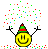# Photo-current and Intensity of light

## Homework Statement

If the frequency and intensity of a light source are both doubled, show that the saturation photo-current remains almost the same.

2. Relevant Graph
https://cnx.org/resources/e73bf218926bd39de4a52bafe3a82d04bbf73542/CNX_UPhysics_39_02_photoexp1.jpg

## The Attempt at a Solution

I am a high-school student. And I have not learnt any relation/equation between saturation photo-current and intensity.
I just know that on increasing the intensity of light, only the no. of photons emitted per unit time over a unit area, increases.
Thus, the no. of photons striking the metal surface increases.
Which in turn increases the no. of electrons coming out of the metal plate, thereby increasing the photo-current.
This thing is also observed experimentally, and is illustrated in the above link of the graph-image.

So i am not getting how the photo-current remains almost the same.
Thank youkuruman
Homework Helper
Gold Member
2021 Award
You have only addressed half of the question. What effect does doubling the frequency have?

I just know that on increasing the intensity of light, only the no. of photons emitted per unit time over a unit area, increases.

Is increasing the number of photons the only way to have higher intensity? Define intensity and I think you’ll see what you are missing here.

You have only addressed half of the question. What effect does doubling the frequency have?
This is the full question that i encountered.
I think both the things, doubling of intensity and doubling of frequency, should effect the saturation photocurrent and cancel each other's effects for almost the same photocurrent.
But I am not getting how?

Is increasing the number of photons the only way to have higher intensity? Define intensity and I think you’ll see what you are missing here.
Definition: Intensity is like brightness, and is measured as the rate at which light energy is delivered to a unit of surface, or energy per unit time per unit area.
Now in photoelectric effect, we only talk about the particle behavior of light.
So in that sense, more intensity means more no. of photons striking a unit area in unit time interval ( this will in turn increase the rate at which energy is delivered to that surface).
Thus on doubling the intensity, the no. of photons striking a unit area of metal in unit time interval are doubled, increasing the no. of photon-electron collisions,and hence, the chances of an electron coming out as a photo-electron increases, and hence the photo-current should increase.
Please tell me where I am going wrong.

rate at which light energy is delivered to a unit of surface, or energy per unit time per unit area.

Correct. Light energy, not number of photons. Now can you write “light energy” in terms of number of photons?

Correct. Light energy, not number of photons. Now can you write “light energy” in terms of number of photons?
yeah, E = n(hc/λ)
where n denotes the no. of photons.
Here, energy is dependent on the number of photons.

yeah, E = n(hc/λ)
where n denotes the no. of photons.
Here, energy is dependent on the number of photons.

So you wrote that in terms of wavelength, but of course you can also express the photon energy in terms of frequency. So the intensity is dependent on the number of photons, but also the frequency of those photons. If you recall the problem called for doubling the frequency.

So you wrote that in terms of wavelength, but of course you can also express the photon energy in terms of frequency. So the intensity is dependent on the number of photons, but also the frequency of those photons. If you recall the problem called for doubling the frequency.
Oh yeah!
E=hν
Hence on doubling the frequency of a photon, we will double its energy!
But this will only effect the maximum kinetic energy of the photoelectrons, and not the photocurrent.
For the photocurrent is proportional to the no. of electrons coming out of the metal in unit time, and this no. of electron depends solely on the intensity, ie. the number of photons per unit area per unit time, and not on the energy of the photons, ie. the frequency.
This is what I am taught.Please suggest if i am going wrong.

kuruman
Homework Helper
Gold Member
2021 Award
Hence on doubling the frequency of a photon, we will double its energy!
Correct. Now suppose that you have two beams of the same intensity (energy per unit area), but the photons in beam 2 have twice the frequency of the photons in beam 1. Which beam has more photons?

•MeAndMyLucidLife
Correct. Now suppose that you have two beams of the same intensity (energy per unit area), but the photons in beam 2 have twice the frequency of the photons in beam 1. Which beam has more photons?
Beam 1 will have more photons!

Okay! Got it! Beam 1 will have twice the number of photons than beam 2!
so firstly on doubling the frequency, we are halving the intensity, and then on doubling the intensity, we end up with almost the same intensity as beginning!
and thus the saturation current remains almost the same!!!

Thanks a lot! Today...You have repaired a great flaw in my understanding...Thank you once again